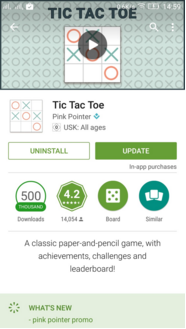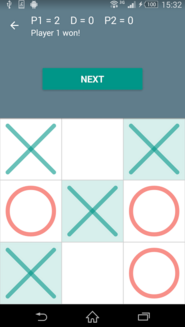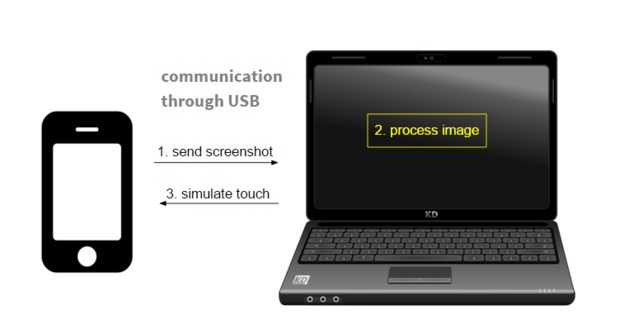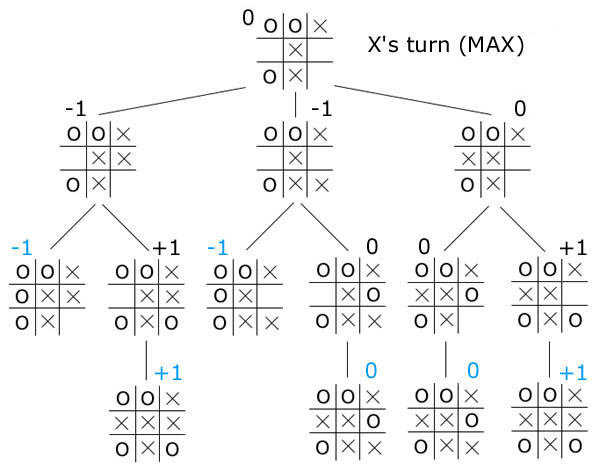## Tic Tac Toe - Automated

### Game Description

This is a two-player game. The player and the system get alternative chances to mark their move on the 3x3 grid provided. One who marks 3 linearly oriented squares first, wins the game. If none of the players can do this, the game ends to be a draw. (The system’s move is marked with a circle and the player’s with a cross. )Difficulty level: Easy

### Overview

The player starts at random cell. The system’s move is identified using Image processing. We are simply calculating intersecting points for blank, O and X for each one of Nine Cell. The further move is calculated by the MinMax algorithm and is marked in the concerned box using ADB Tool. This continues as the algorithm tries to win the game in the best way possible

Get Source Code

### Requirements

• Computer with OpenCV-Python, ADB Tool, Python and required drivers set up.
• An Android Device with the ‘Tic Tac Toe’ game installed on it. (Turn on the Developer options for better visualization)
• USB data transfer cable.

### Block Diagram### Tutorial

Here's the step-wise tutorial to automate the game. The source code is avaiable here.

#### Step 1: Using ADB Tool to capture screenshot

The following command instantaneously takes the screenshot of the connected device and stores it in the SD card following the specified path.

system(' adb shell screencap -p /sdcard/screen.png ');


The following command pulls it from the SD card of the android device into the working system following the path specified.

system(' adb pull /sdcard/screen.png ');


The pulled image is stored in the form of a matrix of pixel values by the MATLAB.

#### Step 2: Image processing

Once the screenshot is obtained, nine different points in the 9 boxes are chosen and processed as blank, O or X. For this game blank cell Red color value is - 255 (RGB) , O's cell R value is - 105 and X's cell R value is - 248.

Here 0 -> blank, 1 -> system's move and 2 -> player.

Now game board is updated with current move. Game was tested on only device Moto G3 (1280x720), for this device intersection of three types of cell is calculated using pixel-color information of screenshot. It is valid for any device with this resolution.

#### Step 3: Algorithm

Minimax is a decision rule used in decision theory, game theory, statistics and philosophy for minimizing the possible loss for a worst case (maximum loss) scenario. Originally formulated for two-player zero-sum game theory, covering both the cases where players take alternate moves and those where they make simultaneous moves, it has also been extended to more complex games and to general decision-making in the presence of uncertainty.###### Algorithm Complexity
Time Complexity: O(b^d), where b is no. of blank cell and d is the depth to which algo. goes
Space Complexity: O(bd)

def min_max_move(instance, marker):
bestmove = None
bestscore = None

if marker == 2:
for m in instance.get_free_cells():
instance.mark(m, 2)
if instance.is_gameover():
score = instance.get_score()
else:
mov_pos, score = min_max_move(instance, 1)
instance.revert_last_move()

if bestscore == None or score > bestscore:
bestscore = score
bestmove = m
else:
for m in instance.get_free_cells():
instance.mark(m, 1)
if instance.is_gameover():
score = instance.get_score()
else:
mov_pos, score = min_max_move(instance, 2)
instance.revert_last_move()

if bestscore == None or score < bestscore:
bestscore = score
bestmove = m
return bestmove, bestscore


#### Step 4: Using ADB Tool to simulate touch

from the result of algorithm tap position is calculated.

The following command taps at the point on the screen with the co-ordinates mentioned as (x, y). This is used to simulate touch at the appropriate points where we want to make a move.

system(' adb shell input tap x y ');


#### Step 5: Testing

After setting up environment, Open game and run script tictactoe.py .

    python tictactoe.py